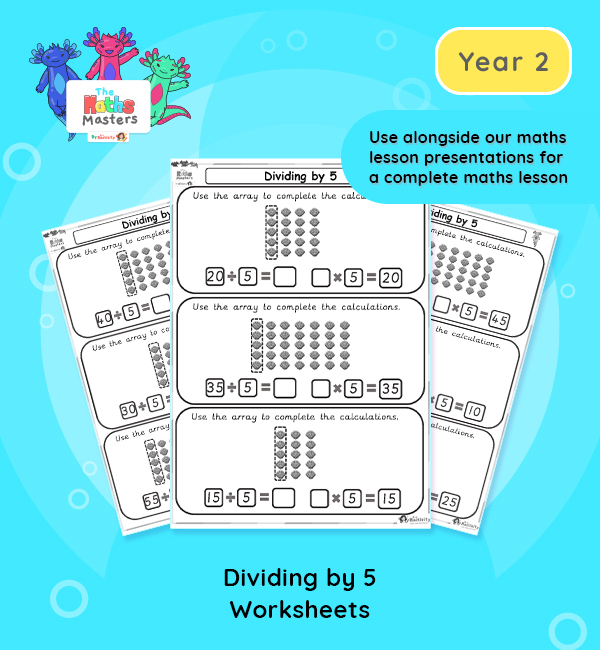# Year 2 | Dividing by 5 Worksheets## Year 2 division worksheets

Aligned with the maths mastery approach, these Year 2 | Dividing by 5 Worksheets are designed to be used alongside our Year 2 | Dividing by 5 Lesson Presentation for a complete maths lesson. The maths worksheets require children to use their knowledge of the 5 times table to work out division problems.

Small Steps: Divide by 5.

NC Links: Recall and use multiplication and division facts for the 2, 5, and 10 times tables. *Calculate mathematical statements for multiplication and division within the multiplication tables and write them using the multiplication, division and equals signs. *Solve problems involving multiplication and division using materials, arrays, repeated addition, mental methods and multiplication and division facts including problems in context. *Count in steps of 2, 3, 5, and 10.

TAF Statements: Working At  – Recall and use multiplication and division facts for 2, 5, and 10 and use them to solve simple problems.

Greater Depth – Recall and use multiplication and division facts for 2, 5, and 10 and make deductions outside known multiplication facts. *Use reasoning about number and relationships to solve more complex problems and explain their thinking.

Ready-to-progress criteria:  2MD-2 Relate grouping problems where the number of groups is unknown to multiplication equation with a missing factor and two division equations (quotitive division)

Year 1 Conceptual prerequisites: Count in multiples of 2, 5, and 10 to find how many groups of 2, 5 or 10 there are in a particular quantity, set in everyday contexts.

## Recently Viewed